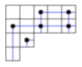## 18.312      Algebraic Combinatorics

 M.I.T. Spring 2002 URL: http://www-math.mit.edu/~apost/courses/18.312/ Instructor: Alexander Postnikov E-mail: apost at math    Office: 2-389
 Lectures: Tuesday, Thursday 1:00-2:30Room 2-102 Office hours: TR 4-5pm, Room 2-389

Course description from the MIT Catalog:

Applications of algebra to combinatorics and conversely. Topics include enumeration methods, partially ordered sets and lattices, matching theory, partitions and tableaux, algebraic graph theory, and combinatorics of polytopes.

• Lecture 1 (02/05/02): Catalan numbers
• [EC2] pp. 219-229, 256-265. This can be found on the web at "Exercises on Catalan and related numbers" (PostSctipt  PDF). See also "Catalan addendum" (PostScript  PDF).

• Lecture 2 (02/07/02): Pattern avoidance in permutations, Young tableux, Schensted correspondence, longest increasing subsequences
• [TAC] Section 8 "A glimpse of Young tableaux".
• C. Schensted "Longest increasing and decreasing subsequences" Canadian Journal of Mathemetics 13 (1961), 179-191. (A copy is available upon request.)

• Lecture 3 (02/12/02): q-Binomial coefficients
• [TAC] Section 6 "Young diagrams and q-binomial coefficients".
• [CC] Section 24 "Gaussian numbers and q-analogues"
• [EC1] Section 1.3 "Permutation statistics"

• Lecture 4 (02/14/02): Labelled trees, Prufer's codes, increasing trees
• [CC] Section 2 "Trees"
• [EC1] Section 1.3 "Permutation statistics"

• Lecture 5 (02/21/02): Symmetric group, statistics on permutations
• [EC1] Section 1.3 "Permutation statistics"

• Lecture 6 (02/26/02): Posets, lattices, distributive lattices, Young's lattice
• [EC1] Sections 3.1 -- 3.4

• Lecture 7 (02/28/02): Up and Down operators, unimodality of Gaussian coefficients.
• [TAC] Section 6 "Young diagrams and q-binomial coefficients"
and Section 8 "A glimpse of Young tableux".

• Lecture 8 (03/05/02): Sperner's and Dilworth's theorems
• [CC] Section 6 "Dilworth's theorem and extremal set theory"
• [TAC] Section 4 "The Sperner property"

• Lecture 9 (03/07/02): De Bruijn sequences
• [CC] Section 8 "De Bruijn sequences"

• Lecture 10 (03/12/02): Partitions: Euler's pentagonal theorem, Jacobi triple product
• [CC] Section 15 "Partitions"

• Lecture 11 (03/14/02): Lindstrom lemma (Gessel-Viennot method). Exponential formula
• [EC2] Section 5.1 "Exponential formula"

• Lecture 12 (03/19/02): Review of Problem Sets 1 and 2

• Lecture 13 (03/21/02): Plane partitions, rombus tilings of hexagon, pseudoline arrangements
• [EC2] Section 7.21 "Plane partitions with bounded part size"

• Lecture 14 (04/02/02): Spanning trees, greedy algorithm, Matrix-Tree theorem
• [CC] Section 2 "Trees"
• [CC] Section 34 "Electrical networks and squared matrices"

• Lecture 15 (04/04/02): Matrix-Tree theorem (cont'd)
• [CC] Section 34 "Electrical networks and squared matrices"

• Lecture 16 (04/09/02): Review of Problem Set 3.

• Lecture 17 (04/11/02): Weighted lattice paths and continued fractions
• I.P.Goulden, D.M.Jackson: Combinatorial Enumeration, John Wiley & Sons, 1983
Section 5 "Combinatorics of Paths"

• Lecture 18 (04/18/02): Electrical networks
• [TAC] Section 11.3 "Electrical networks"
• [CC] Section 34 "Electrical networks and squared squares"

• Lecture 19 (04/23/02): Chromatic polynomial, acyclic orientations, Mobius function
• [CC] Section 25 "Lattices and Mobius inversion"

• Lecture 20 (04/25/02): Permutohedra, Newton polytopes, zonotopes

• Lecture 21 (04/30/02): Birkhoff polytope and Hall's marriage theorem.
• [CC] Section 5 "Systems of distinct representatives

• Lecture 22 (05/02/02): Domino tilings of rectangles

• Lecture 23 (05/07/02): TBA

• Lecture 24 (05/09/02): Guest lecture by James Propp

• Lecture 25 (05/14/02): TBA
Recommended Texts: (The students are not required to have these books.)

[TAC] Topics in Algebraic Combinatorics, Course notes by R. P. Stanley for Mathematics 192 (Algebraic Combinatorics), Harvard University, Fall 2000.   PostScript   PDF

[CC] A course in Combinatorics by J. H. van Lint and R. M. Wilson, Cambridge University Press, 1992 (reprinted 1994, 1996).

[EC1] Enumerative Combinatorics, Vol 1 by R. P. Stanley, Wadsworth and Brooks/Cole, Pacific Grove, CA, 1986; second printing, Cambridge University Press, 1996.

[EC2] Enumerative Combinatorics, Vol 2 by R. P. Stanley, Cambridge University Press, 1999.

Problem Sets

Tentative List of Topics:

• Catalan Numbers, Triangulations, Catalan Paths, Noncrossing Partitions
• Walks in Graphs, Random Walks on the n-Cube, Spectra of Graphs
• Symmetric Group, Statistics on Permutations, Inversions and Major Index
• Partially Ordered Sets and Lattices, Sperner's Theorem
• q-Binomial Coefficients, Gaussian coefficients, and Young Diagrams
• Young's Lattice, Tableaux, and Schensted's Correspondence
• Trees, Parking Functions, and Prufer Codes
• Matrix-Tree Theorem, Electrical Networks
• Enumeration under Group Action, Burnside's Lemma, Polya Theory
• Transportation and Birkhoff Polytopes, Cyclic Polytopes, Permutohedra
• Matching Enumeration, Pfaffians, Ising Model, Domino Tilings